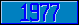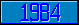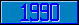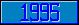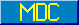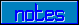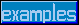## \$%LOG

### M[UMPS] by Example

```
2-Jul–96, 6:54:35

Routine Save for General Mathematics Library function \$%LOG^MATH

;

; Unless otherwise noted, the code below

; was approved in document X11/95–11

;

; If corrections have been applied,

; first the original line appears,

; with three semicolons at the beginning of the line.

;

; Then the source of the correction is acknowledged,

; then the corrected line appears, followed by a

; line containing three semicolons.

;

LOG(X,PREC) ;

New L,LIM,M,N,K,VALUE

If X'>0 Set \$Ecode=",M28,"

Set PREC=\$Get(PREC,11)

Set M=1

;

;;; If X>0 For N=0:1 Quit:(X/M)<10  Set M=M*10

; Winfried Gerum (8 June 1995)

For N=0:1 Quit:(X/M)<10  Set M=M*10

;;;

;

If X<1 For N=0:–1 Quit:(X/M)>0.1  Set M=M*0.1

Set X=X/M

Set X=(X–1)/(X+1),(VALUE,L)=X

Set LIM=\$Select((PREC+3)'>11:PREC+3,1:11),@("LIM=1E-"_LIM)

For K=3:2 Set L=L*X*X,M=L/K,VALUE=M+VALUE Set:M<0 M=-M Quit:M<LIM

Set VALUE=VALUE*2+(N*2.30258509298749)

Quit VALUE

;===

;

;

;

```